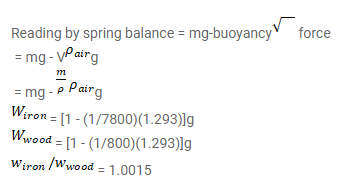# Find the ratio of the weights, as measured by a spring balance,

Question:

Find the ratio of the weights, as measured by a spring balance, of a $1 \mathrm{~kg}$ block of iron and a $1 \mathrm{~kg}$ block of wood. Density of iron $=7800 \mathrm{~kg} / \mathrm{m}^{3}$, density of wood $=800 \mathrm{~kg} / \mathrm{m}^{3}$ and density of air $=1.293 \mathrm{~kg} / \mathrm{m}^{3}$.

Solution: